## Saturday, December 28, 2013

### HP Prime: Drawing Pixelated Pictures (DRAWICON4, DRAWICON6)

Drawing Iconic Pictures with the HP Prime

4 x 4 Pixels: DRAWICON4(number)

Entry: Real number, integer

The number is ultimately converted into a binary number, with each of the 16 bits fitting a 4 x 4 matrix. If the square is turned "on" (having a value of 1), a square will be drawn. Each space if the matrix represents a binary bit: 2^0, 2^1, 2^2, 2^3, etc. The lowest value bit is located at the bottom, right-hand corner element of the matrix.

You can specify whether the squares will be drawn in black or a specific color. The 4 x 4 matrix of bits is represented as this:

[ [ 2^15, 2^14, 2^13, 2^12 ],
[ 2^11, 2^10, 2^9, 2^8 ],
[ 2^7, 2^6, 2^5, 2^4 ],
[ 2^3, 2^2, 2^1, 2^0 ] ]

Program:

Comments followed by // are for notes and do not necessarily have to be typed.

EXPORT DRAWICON4(m)
BEGIN
// EWS 12/28/2013

// does the user want color?
LOCAL ch, R, G, B;
CHOOSE(ch,"Color?","Black","Yes");
IF ch==1 THEN
ch:=RGB(0,0,0);
ELSE
INPUT({R,G,B}, "Color Input",
{"Red","Green","Blue"},{ },{0,0,0});
ch:=RGB(R,G,B);
END;

// convert m to real to be broken down
// Type 1 is an integer (#)
IF TYPE(m)==1 THEN
m:=B→R(m);
END;

// remove negation and fraction parts (so entries like -12 and 34.55 are acceptable)
m:=ABS(IP(m));

// allow m to be text by storing it in M
LOCAL M:=m;

// check for upper limit
IF m>65535 THEN
MSGBOX("Excess of 65535!");
KILL;
END;

// break m into its binary parts and puts them in the required order
LOCAL n:=15,r,c,
mat:=MAKELIST(0,X,1,16);

REPEAT
IF 2^n≤m THEN
m:=m-2^n;
mat(n+1):=1;
END;
n:=n-1;
UNTIL n<0;
mat:=REVERSE(mat);

// list2mat(list, number of columns) - found in the catalog
mat:=list2mat(mat,4);

// drawing a border and label
RECT();
RECT_P(129,79,189,139,RGB(0,0,255),
RGB(255,255,255));
TEXTOUT_P(M,139,0,4,RGB(0,128,0));

// use the matrix to draw the squares
// pixels x = columns, y = rows
FOR r FROM 1 TO 4 DO
FOR c FROM 1 TO 4 DO

IF mat(r,c)==1 THEN
RECT_P(129+15*(c-1), 79+15*(r-1),
129+15*c, 79+15*r, ch);
END;
END;
END;

WAIT(0);

END;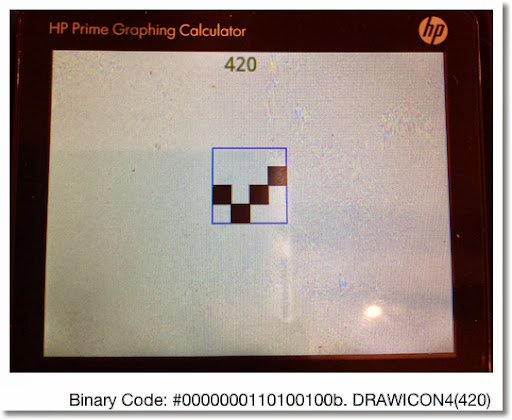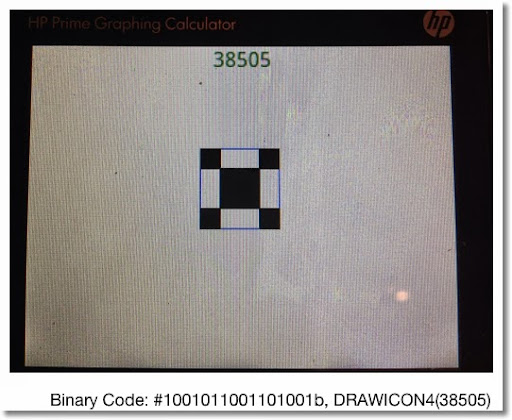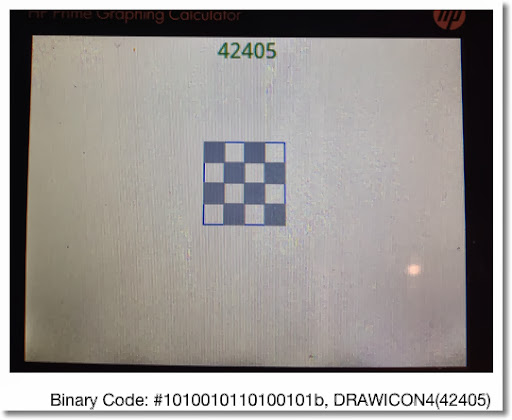Here is a 6 x 6 version:

DRAWICON6

6 x 6 Pixels: DRAWICON6(number)

Entry: Real number, integer

The program is an almost carbon-copy of DRAWICON4, except the limits have changed.

Program:

Comments followed by // are for notes and do not necessarily have to be typed.

EXPORT DRAWICON6(m)
BEGIN
// EWS 12/28/2013

// does the user want color?
LOCAL ch, R, G, B;
CHOOSE(ch,"Color?","Black","Yes");
IF ch==1 THEN
ch:=RGB(0,0,0);
ELSE
INPUT({R,G,B}, "Color Input",
{"Red","Green","Blue"},{ },{0,0,0});
ch:=RGB(R,G,B);
END;

// convert m to real to be broken down
// Type 1 is an integer (#)
IF TYPE(m)==1 THEN
m:=B→R(m);
END;

// remove negation and fraction parts (so entries like -12 and 34.55 are acceptable)
m:=ABS(IP(m));

// allow m to be text by storing it in M
LOCAL M:=m;

// check for upper limit
IF m>2^36-1 THEN
MSGBOX("Excess!");
KILL;
END;

// break m into its binary parts and puts them in the required order
LOCAL n:=35,r,c,
mat:=MAKELIST(0,X,1,36);

REPEAT
IF 2^n≤m THEN
m:=m-2^n;
mat(n+1):=1;
END;
n:=n-1;
UNTIL n<0;
mat:=REVERSE(mat);

// list2mat(list, number of columns) - found in the catalog
mat:=list2mat(mat,6);

// drawing a border and label
RECT();
RECT_P(129,79,219,169,RGB(0,0,255),
RGB(255,255,255));
TEXTOUT_P(M,139,0,4,RGB(0,128,0));

// use the matrix to draw the squares
// pixels x = columns, y = rows
FOR r FROM 1 TO 6 DO
FOR c FROM 1 TO 6 DO

IF mat(r,c)==1 THEN
RECT_P(129+15*(c-1), 79+15*(r-1),
129+15*c, 79+15*r, ch);
END;
END;
END;

WAIT(0);

END;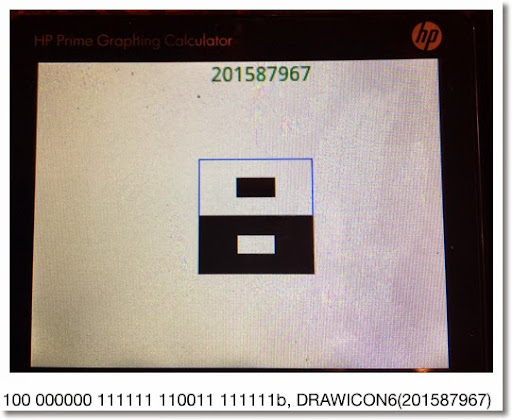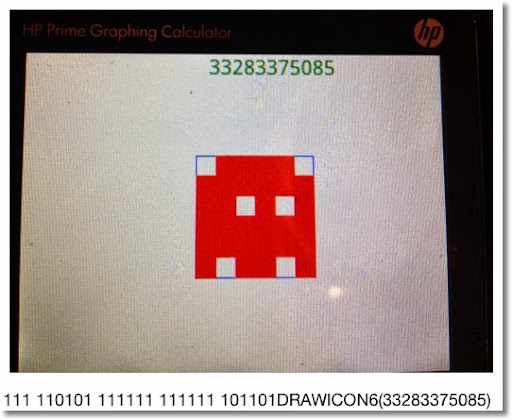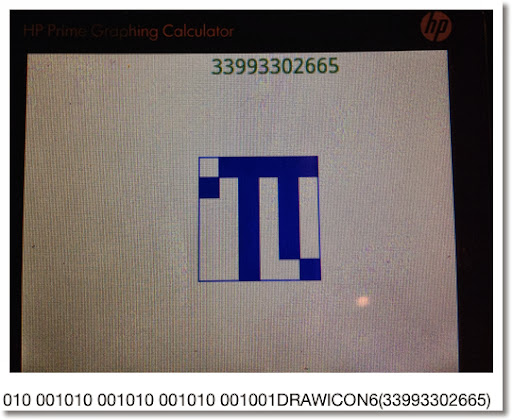Have fun and thank you as always!

I wonder how much of a challenge to do this on a TI-84?

See you next time!

Eddie

This blog is property of Edward Shore. 2013

#### 1 comment:

1.I enjoyed reading this blog post. It was inspiring and informative. Thank you
Sbobet777 login, Online Slot. Joker688. Joker138, Joker Slot APK

### Swiss Micros DM16L: Advanced Boolean and Factorial (up to 20)

Swiss Micros DM16L:   Advanced Boolean and Factorial (up to 20) Introduction The program listing, for the Swiss Micros DM16L and Hewlett Pac...